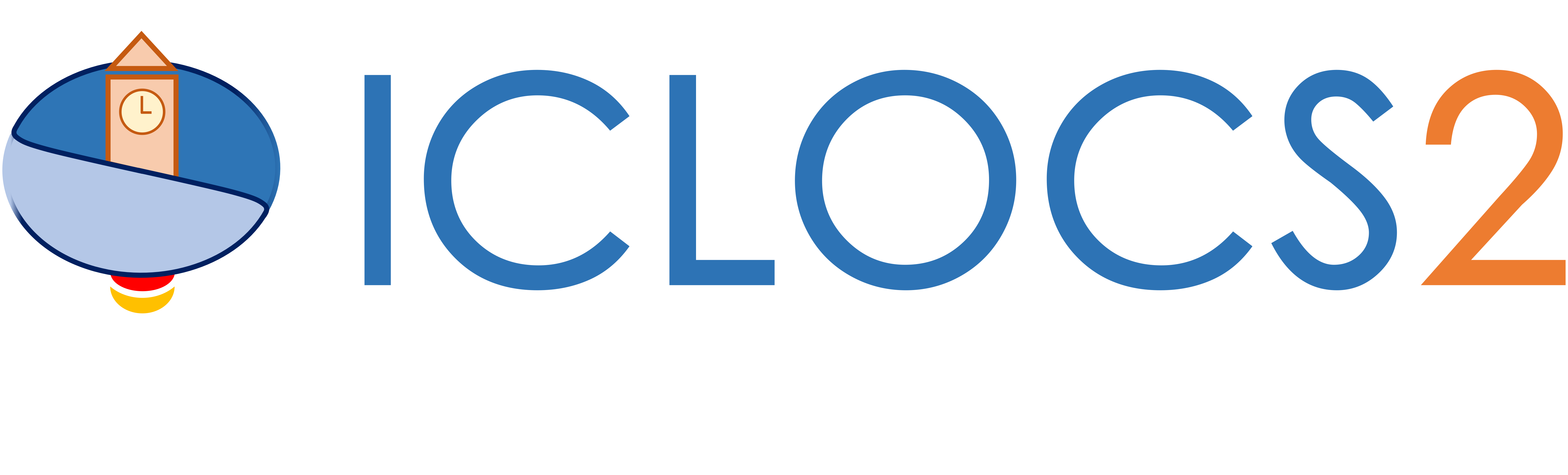Get Started

Solving a multi-phase problem

This guide will help you setup the solve of a multi-phase optimal control problem with main.m.

Fetch, transcribe and solve the problem

First we load the formulated optimal control problem (in myMultiPhaseProblem.m)

[problem,guess,options.phaseoptions]=myMultiPhaseProblem;

Then we can load the corrsponding options and solver settings.

options.mp= settings_myMultiPhaseProblem;

Next, the problem can be transcribed and solved using the following standard command

[solution,MRHistory]=solveMyProblem( problem,guess,options);

with the returning structure variable solution containing the raw output from the NLP solver (sampled data points), the represented continous time solution and outcomes of error analysis.

Optional functions

Generation of figures

In ICLOCS 2.5, various figures can be generated for the multiphase problem with a single line of command

genSolutionPlots(options, solution);

The type of plots to be generated is spcified in the settings file with options.plot having the values

• 1: Plot all figures (state and input trajectory, multipliers/costate values and errors)
• 2: Plot only the state and input trajectory
• 3: Plot only the multipliers/costate values
• 4: Plot only the error values (absolute local error, relative local error and absolute constraint violation error)

Solution variables

The solution variable for a multi-phase problem contains two variables: mp collects the solutions related to the overall problem, and phaseSol contains the solution variables for each phases. Here we provide short descriptions for some of them.

• multipliers: a structure variable containing the multiplier/costate information
• computation_time: time spent on solving the NLP problem
• z: the NLP variable vector returned from the NLP solver
• t0: the initial time
• tf: the terminal(final) time
• p: parameters values (part of optimization solution)
• X: sampled state values at mesh points
• x0: initial states
• U: sampled input/control values at mesh points
• T: a time array corresponding to the mesh points
• z_orgscale: the NLP variable vector after transforming back to the original variable scale
• multipliers: a structure variable containing information at all collocation points (for Hermite-Simpson method)
• Xp: Constructed continuous state trajectory
• dXp: Constructed continuous state rate (dynamics) trajectory
• Up: Constructed input/control trajectory
• Error: the absolute local error for state variables
• ErrorRelative: the relative local error for state variables
• ConstraintError: the absolute constraint violation error (organized as [path constraint upper bound, path constraint lower bound, state upper bound, state lower bound, input upper bound, input lower bound]
• T_ConstraintError: a time array corresponding to the absolute constraint violation error evaluation
• NumActiveConstraint: the number of active constraints
• TSeg_Bar: time interval barriers between LGR polynomial segments (p/hp method only)
• dX: sampled state rate values at mesh points
• dU: sampled control rate values at mesh points

In the mesh refinement history variable MRHistory, we have

• errorHistory: history of absolute local errors
• timeHistory: NLP solving time for each MR iteration
• iterHistory: number of NLP iterations for each MR iteration
• ConsraintErrorHistory: history of constraint violation errors
• resErrorHistory: history of integrated residual errors
• statusHistory: history of NLP exit flags
• solutionHistory: solution of each MR iteration

Evaluation of contructed solution

The constructed continuous trajectoris (Xp, dXp and Up) are in the form of piecewise splines, and can be evaluated in the following ways.

Suppose we want to obtain the values of the state at 1000 equally spaced points along the trajectory of phase i

sol=solution.phaseSol{i};
tt=linspace(sol.t0,sol.tf,1000);

The command for obtaining the corrsponding values of the different state and input variable will be

x1=speval(sol,'X',1,tt);
x2=speval(sol,'X',2,tt);
...
u1=speval(sol,'U',1,tt);
u2=speval(sol,'U',2,tt);
...